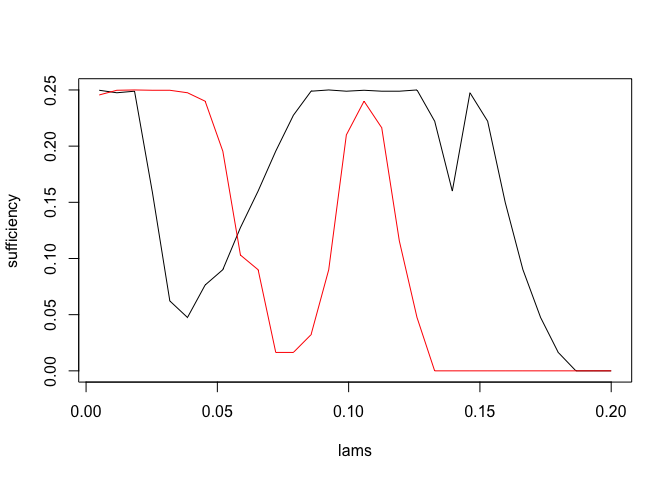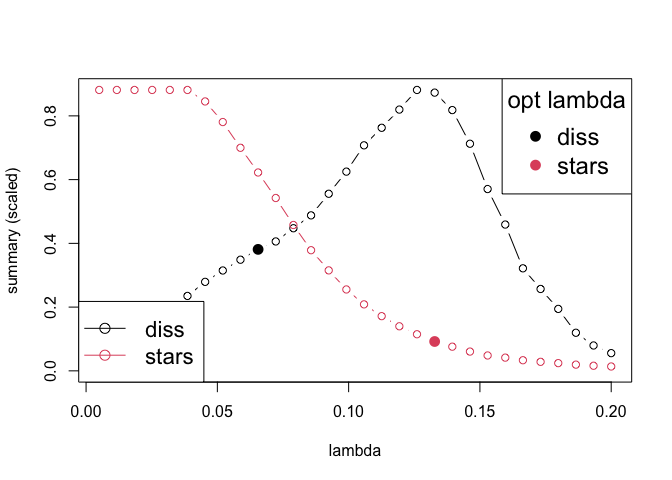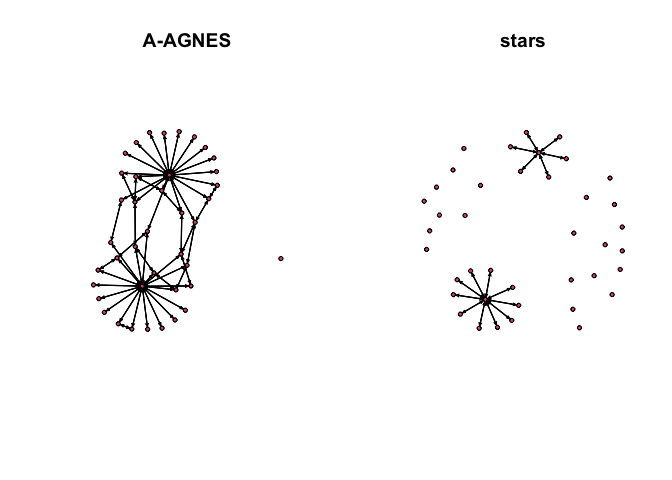# Other selection criteria with pulsar

In addition to StARS’ edge stability and G-StARS’ induced subgraph stability, pulsar can be used to compute other model selection criteria. By defining a few auxiliary functions, pulsar can conveniently recapitulate a number of recently proposed selection criteria, e.g., Tandon and Ravikumar (2014)’s sufficiency measure for hub discovery or Caballe, Bochkina, and Mayer (2015)’s augmented AGNES scheme.

## Learning Graphs with a few hubs

The sufficiency criterion defined by Tandon and Ravikumar (2014) uses edge stability to identify hub and non-hub nodes. Graphs are learned from the neighborhoods of non-hub nodes only, but, since hub nodes neighbor non-hubs, an entire graph can be learned more accurately with fewer samples. The paper includes sample complexity bounds for Ising graphical models. In this example, we generate correlated binary data for cheap (compared to Gibbs sampling) with Normal copula functions.

  library(huge)
library(Matrix)

p <- 40
n <- round(8*p * log(p))

set.seed(10010)
dat <- huge.generator(n, p, 'hub', verbose=FALSE, v=.3, u=.1)

## Generate correlated binomial data with the Normal copula method
X  <- apply(apply(scale(dat$data), 2, pnorm), 2, qbinom, size=1, prob=.5) ising.net <- function(Z, lambda, link='binomial') { p <- ncol(Z) l <- length(lambda) estFun <- function(i) { betamat <- matrix(NA, p, l) betamat[-i,] <- as.matrix(glmnet::glmnet(Z[,-i], Z[,i], family=link, lambda=lambda)$beta)
betamat
}
est <- parallel::mcmapply(estFun, 1:p, mc.cores=1, SIMPLIFY='array')
list(path=apply(est, 2, function(x) { diag(x) <- 0 ; as(x!=0, "lgCMatrix") }))
}

lams <- getLamPath(.2, .005, 30)
out  <- pulsar(X, ising.net, fargs=list(lambda=lams), criterion=c('stars', 'sufficiency'),
subsample.ratio=.6, rep.num=60, seed=10010)
## Warning: 1 job had warning: "as(<matrix>, "lgCMatrix") is deprecated since
## Matrix 1.5-0; do as(as(as(., "lMatrix"), "generalMatrix"), "CsparseMatrix")
## instead"

For non-hubs, the sufficiency metric should have a large dip in the regularization path while hub nodes are expected to be relatively flat:

plot(lams, out$sufficiency$merge[1,], type='l', ylab="sufficiency")
points(lams, out$sufficiency$merge[4,], type='l', col='red')Estimate the hub graph by excluding hub nodes from neighborhood selection (algorithm 2 from the paper)

  tandonest <- function(i, out, tu, tl) {
rmerge <- out$sufficiency$merge
p <- nrow(rmerge)
l <- ncol(rmerge)
prime  <- tail(which(rmerge[i,] > tu), 1)
if (length(prime) == 0) return(rep(FALSE, p))
naught <- tail(which(rmerge[i,1:prime] < tl), 1)
if (length(naught) == 1) {
pmerge <- out$stars$merge[[naught]][i,]
return(pmerge >= (1+sqrt(1-4*tl))/2)
} else return(rep(FALSE, p))
}

net <- sapply(1:p, tandonest, out=out, tu=.2, tl=.15)
## Symmetrize
net <- sign(t(net) + net)

## Augmented AGNES

To replicate the augmented AGNES (A-AGNES) method of Caballe, Bochkina, and Mayer (2015), use the node-wise dissimilarity metric (diss) and the AGNES algorithm as implemented in the cluster package. A-AGNES selects the lambda that minimizes the variance of the estimated diss + the [squared] bias of the expected estimated dissimilarities w.r.t. the AGNES-selected graph - that has the maximum agglomerative coefficient over the path.

dat <- huge.generator(n, p, 'hub', verbose=FALSE, v=.1, u=.4)
out.diss  <- pulsar(dat$data, fargs=list(lambda=lams, verbose=FALSE), rep.num=20, criterion=c('diss', 'stars')) fit <- refit(out.diss, 'stars') ## Compute the max agglomerative coefficient over the full path path.diss <- lapply(fit$est$path, pulsar:::graph.diss) library(cluster) acfun <- function(x) agnes(x, diss=TRUE)$ac
ac <- sapply(path.diss, acfun)
ac.sel <- out.diss$diss$merge[[which.max(ac)]]

## Estimate the diss bias
dissbias <- sapply(out.diss$diss$merge,
function(x) mean((x-ac.sel)^2)/2)
varbias  <- out.diss$diss$summary + dissbias

## Select the index and refit
opt.index(out.diss, 'diss') <- which.min(varbias)
fit.diss <- refit(out.diss)

plot(out.diss)par(mfrow=c(1,2))
plot(network::network(as.matrix(fit.diss$refit$diss)), main='A-AGNES')
plot(network::network(as.matrix(fit.diss$refit$stars)), main='stars')